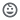# Edu Game

10+Everyone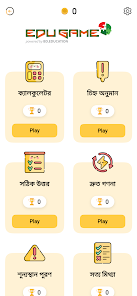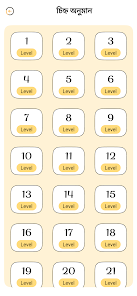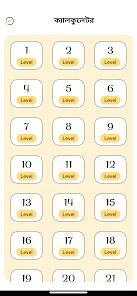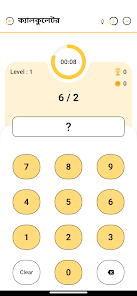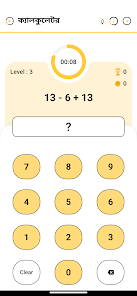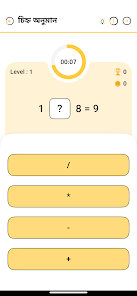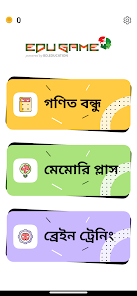Edu Game is actually a math puzzle for Children to learn addition, subtraction, multiplication and division. It contains various scopes to make their brains more intellectual.

Want to improve your math skills of children? ❓ How about helping your Children master mathematics with fun, free Edu Games? ✔️ Edu Games is the perfect way to help children learn math skills the easy way! 👍

Our Edu Games for Children is super fun! Solve a wide variety of math puzzles, brain teasers, and brain math puzzles using nothing more than basic arithmetic. Pick up new skills in addition ➕, subtraction ➖, multiplication ✖️, and division, ➗ or get more advanced with fractions ¼, decimals •, and mixed operations.

📚 Learn from all the fun free educational modes below:
◾ Subtraction games - 1, 2, 3 digit subtraction game to learn how to subtract
◾ Multiplication games - Best practice game to learn multiplication tables and multiplying methods.
◾ Division games - Learn to divide by playing multiple fun division games
◾ Fractions - Step-by-step learning of fraction calculation, fun and easy way to learn fractions.
◾ Decimals - Fun adding, subtracting, multiplying and dividing decimal modes to learn
◾ Square Roots - Practice squares and square roots, learn how to squares a number
◾ Exponents - Practice exponent problems
◾ Mixed operations - Put your knowledge to the test by practicing addition, subtraction, multiplication, division all in one mode!

Test your addition, subtraction, multiplication, and other number skills in the following modes:
🎴 Memory Match - Flip number memory cards and match answers to the equations.
⏲️ Challenge Mode - Finish the puzzles before time runs out!
👫 Dual Mode - Split-screen interface for two players.

Edu Games for Children should be fun! ✔️ Our math app is suitable for children in kindergarten, 1st grade, 2nd grade, 3rd grade, 4th grade, 5th grade, or 6th grade, and of course, any teenager or adult who is interested in training their brain and improving their math skills! ✏️

Updated on
Jan 29, 2023

## Data safety

Safety starts with understanding how developers collect and share your data. Data privacy and security practices may vary based on your use, region, and age. The developer provided this information and may update it over time.No data shared with third parties